# C++的STL总结（1）

2019/01/24 23:12

没有很系统的学过算法，c++也只是学些基础，虽然经常会用一些STL里面的函数，但是并没有对STL模板库有一个清晰的了解，趁着寒假有时间就自己在网上百度浏览别人的总结的内容，自己汇集并总结了一下，希望对自己以后的路能有所帮助。

一：STL简介

STL（Standard Template Library，标准模板库)是惠普实验室开发的一系列软件的统称。它是由Alexander Stepanov、Meng Lee和David R Musser在惠普实验室工作时所开发出来的。现在虽说它主要出现在C++中，但在被引入C++之前该技术就已经存在了很长的一段时间。STL的代码从广义上讲分为三类：algorithm（算法）、container（容器）和iterator（迭代器），几乎所有的代码都采用了模板类和模版函数的方式，这相比于传统的由函数和类组成的库来说提供了更好的代码重用机会。在C++标准中，STL被组织为下面的13个头文件<algorithm>、<deque>、<functional>、<iterator>、<vector>、<list>、<map>、<memory>、<numeric>、<queue>、<set>、<stack>和<utility>。

二：容器

在实际的开发过程中，数据结构本身的重要性不会逊于操作于数据结构的算法的重要性，当程序中存在着对时间要求很高的部分时，数据结构的选择就显得更加重要。经典的数据结构数量有限，但是我们常常重复着一些为了实现向量、链表等结构而编写的代码，这些代码都十分相似，只是为了适应不同数据的变化而在细节上有所出入。STL容器就为我们提供了这样的方便，它允许我们重复利用已有的实现构造自己的特定类型下的数据结构，通过设置一些模版类，STL容器对最常用的数据结构提供了支持，这些模板的参数允许我们指定容器中元素的数据类型，可以将我们许多重复而乏味的工作简化。

容器部分主要由头文件<vector>,<list>,<deque>,<set>,<map>,<stack>和<queue>组成。对于常用的一些容器和容器适配器（可以看作由其它容器实现的容器），可以通过下表总结一下它们和相应头文件的对应关系。

三：迭代器

概括来说，迭代器在STL中用来将算法和容器联系起来，起着一种黏和剂的作用。几乎STL提供的所有算法都是通过迭代器存取元素序列进行工作的，每一个容器都定义了其本身所专有的迭代器，用以存取容器中的元素。迭代器部分主要由头文件<utility>,<iterator>和<memory>组成。

四：常用库函数总结

（1）C++迭代器

正文

迭代器是一种检查容器内元素并遍历元素的数据类型。C++更趋向于使用迭代器而不是下标操作，因为标准库为每一种标准容器（如vector）定义了一种迭代器类型，而只用少数容器（如vector）支持下标操作访问容器元素。

定义和初始化：

每种容器都定义了自己的迭代器类型，如vector:

``vector<int>::iterator    iter;    //定义一个名为iter的变量``

每种容器都定义了一对名为begin和en的函数，用于返回迭代器。下面对迭代器进行初始化操作：

``````vector<int>    ivec;
vector<int>::iterator    iter1=ivec.bengin();    //将迭代器iter1初始化为指向ivec容器的第一个元素

vector<int>::iterator   iter2=ivec.end();    //将迭代器iter2初始化为指向ivec容器的最后一个元素的下一个位置``````

注意end并不指向容器的任何元素，而是指向容器的最后元素的下一位置，称为超出末端迭代器。如果vector为空，则begin返回的迭代器和end返回的迭代器相同。一旦向上面这样定义和初始化，就相当于把该迭代器和容器进行了某种关联，就像把一个指针初始化为指向某一空间地址一样。

常用操作

``````1 *iter                //对iter进行解引用，返回迭代器iter指向的元素的引用
2 iter->men            //对iter进行解引用，获取指定元素中名为men的成员。等效于(*iter).men
3 ++iter                //给iter加1，使其指向容器的下一个元素
4 iter++
5 --iter                //给iter减1，使其指向容器的前一个元素
6 iter--
7 iter1==iter2        //比较两个迭代器是否相等，当它们指向同一个容器的同一个元素或者都指向同同一个容器的超出末端的下一个位置时，它们相等
8 iter1!=iter2``````

假设已经声明一个vector<int>的ivec容器，下面用迭代器来遍历ivec容器，把其每个元素重置为0：

1 for(vector<int>::iterator iter=ivec.begin();iter!=ivec.end();++iter) 2 *iter=0;

在C++定义的容器类型中，只有vector和queue容器提供迭代器算数运算和除!=和==之外的关系运算：

``````1 iter+n     //在迭代器上加（减）整数n，将产生指向容器中钱前面（后面）第n个元素的迭代器。新计算出来的迭代器必须指向容器中的元素或超出容器末端的下一个元素
2 iter-n
3
4 iter1+=iter2        //将iter1加上或减去iter2的运算结果赋给iter1。两个迭代器必须指向容器中的元素或超出容器末端的下一个元素
5 iter1-=iter2
6
7 iter1-iter2            //两个迭代器的减法，得出两个迭代器的距离。两个迭代器必须指向容器中的元素或超出容器末端的下一个元素
8
9 >,>=,<,<=        //元素靠后的迭代器大于靠前的迭代器。两个迭代器必须指向容器中的元素或超出容器末端的下一个元素``````

注意两个迭代器相减得出两个迭代器对象的距离，该距离名为difference_type的signed类型的值，该类型类似于size_type类型，也是有vector定义的。可以迭代器算术操作来移动迭代器直接指向某个元素：

1 vector<int>::iterator mid=v.begin()+v.size()/2; //初始化mid迭代器，使其指向v中最靠近正中间的元素

迭代器const_iterator

每种容器还定义了一种名为const_iterator的类型。该类型的迭代器只能读取容器中的元素，不能用于改变其值。之前的例子中，普通的迭代器可以对容器中的元素进行解引用并修改，而const_iterator类型的迭代器只能用于读不能进行重写。例如可以进行如下操作：

``````1 for(vector<int>::const_iterator iter=ivec.begin();iter!=ivec.end();++iter)
2      cout<<*iter<<endl;       //合法，读取容器中元素值
3
4 for(vector<int>::const_iterator iter=ivec.begin();iter!=ivec.end();++iter)
5     *iter=0;        //不合法，不能进行写操作``````

const_iterator和const  iterator是不一样的，后者对迭代器进行声明时，必须对迭代器进行初始化，并且一旦初始化后就不能修改其值。这有点像常量指针和指针常量的关系。例如：

``````1 vector<int>    ivec(10);
2 const    vector<int>::iterator    iter=ivec.begin();
3 *iter=0;    //合法，可以改变其指向的元素的值
4 ++iter;    //不合法，无法改变其指向的位置``````

## 由于一些对容器的操作如删除元素或移动元素等会修改容器的内在状态，这会使得原本指向被移动元素的迭代器失效，也可能同时使其他迭代器失效。使用无效的迭代器是没有定义的，可能会导致和使用悬垂指针相同的问题。所以在使用迭代器编写程序时，需要特别留意哪些操作会使迭代器失效。使用无效迭代器会导致严重的运行时错误。

（2）求和（<numeric> accumulate）

`````` 1 #include <iostream>
2 #include <vector>
3 #include <numeric>
4
5 using namespace std;
6
7 int main()
8 {
9     vector<int> v;
10     for(int i = 0; i < 10; ++i )
11     {
12         v.push_back(i);
13     }
14     for(vector<int>::iterator it = v.begin(); it != v.end(); ++it)
15     {
16         cout << *it << " ";
17     }
18     cout << endl;
19     cout << accumulate(v.begin(),v.end(),0) << endl;
20     return 0;
21 }``````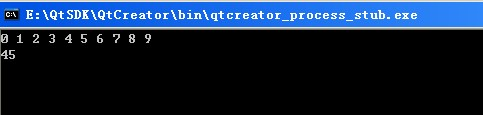（3）vector（动态数组）

vector 是向量类型，它可以容纳许多类型的数据，如若干个整数，所以称其为容器。vector 是C++ STL的一个重要成员，使用它时需要包含头文件：

``#include<vector>;``

一、vector 的初始化：可以有五种方式,举例说明如下：``````(1) vector<int> a(10); //定义了10个整型元素的向量（尖括号中为元素类型名，它可以是任何合法的数据类型），但没有给出初值，其值是不确定的。
（2）vector<int> a(10,1); //定义了10个整型元素的向量,且给出每个元素的初值为1
（3）vector<int> a(b); //用b向量来创建a向量，整体复制性赋值
（4）vector<int> a(b.begin(),b.begin+3); //定义了a值为b中第0个到第2个（共3个）元素
（5）int b={1,2,3,4,5,9,8};
vector<int> a(b,b+7); //从数组中获得初值``````二、vector对象的几个重要操作，举例说明如下：``````（1）a.assign(b.begin(), b.begin()+3); //b为向量，将b的0~2个元素构成的向量赋给a
（2）a.assign(4,2); //是a只含4个元素，且每个元素为2
（3）a.back(); //返回a的最后一个元素
（4）a.front(); //返回a的第一个元素
（5）a[i]; //返回a的第i个元素，当且仅当a[i]存在2013-12-07
（6）a.clear(); //清空a中的元素
（7）a.empty(); //判断a是否为空，空则返回ture,不空则返回false
（8）a.pop_back(); //删除a向量的最后一个元素
（9）a.erase(a.begin()+1,a.begin()+3); //删除a中第1个（从第0个算起）到第2个元素，也就是说删除的元素从a.begin()+1算起（包括它）一直到a.begin()+         3（不包括它）
（10）a.push_back(5); //在a的最后一个向量后插入一个元素，其值为5
（11）a.insert(a.begin()+1,5); //在a的第1个元素（从第0个算起）的位置插入数值5，如a为1,2,3,4，插入元素后为1,5,2,3,4
（12）a.insert(a.begin()+1,3,5); //在a的第1个元素（从第0个算起）的位置插入3个数，其值都为5
（13）a.insert(a.begin()+1,b+3,b+6); //b为数组，在a的第1个元素（从第0个算起）的位置插入b的第3个元素到第5个元素（不包括b+6），如b为1,2,3,4,5,9,8         ，插入元素后为1,4,5,9,2,3,4,5,9,8
（14）a.size(); //返回a中元素的个数；
（15）a.capacity(); //返回a在内存中总共可以容纳的元素个数
（16）a.resize(10); //将a的现有元素个数调至10个，多则删，少则补，其值随机
（17）a.resize(10,2); //将a的现有元素个数调至10个，多则删，少则补，其值为2
（18）a.reserve(100); //将a的容量（capacity）扩充至100，也就是说现在测试a.capacity();的时候返回值是100.这种操作只有在需要给a添加大量数据的时候才         显得有意义，因为这将避免内存多次容量扩充操作（当a的容量不足时电脑会自动扩容，当然这必然降低性能）
（19）a.swap(b); //b为向量，将a中的元素和b中的元素进行整体性交换
（20）a==b; //b为向量，向量的比较操作还有!=,>=,<=,>,<``````三、顺序访问vector的几种方式，举例说明如下：
（1）向向量a中添加元素
1、

``````1  vector<int> a;
2 for(int i=0;i<10;i++)
3 a.push_back(i);``````

2、也可以从数组中选择元素向向量中添加

``````int a={1,2,3,4,5,6};
vector<int> b；
for(int i=1;i<=4;i++)
b.push_back(a[i]);``````

3、也可以从现有向量中选择元素向向量中添加

``````int a={1,2,3,4,5,6};
vector<int> b;
vector<int> c(a,a+4);
for(vector<int>::iterator it=c.begin();it<c.end();it++)
b.push_back(*it);``````

4、也可以从文件中读取元素向向量中添加

``````ifstream in("data.txt");
vector<int> a;
for(int i; in>>i)
a.push_back(i);``````

5、【误区】

``````vector<int> a;
for(int i=0;i<10;i++)
a[i]=i;``````

//这种做法以及类似的做法都是错误的。刚开始我也犯过这种错误，后来发现，下标只能用于获取已存在的元素，而现在的a[i]还是空的对象

（　　2）从向量中读取元素
1、通过下标方式读取

``````int a={1,2,3,4,5,6};
vector<int> b(a,a+4);
for(int i=0;i<=b.size()-1;i++)
cout<<b[i]<<" ";``````

2、通过遍历器方式读取

``````int a={1,2,3,4,5,6};
vector<int> b(a,a+4);
for(vector<int>::iterator it=b.begin();it!=b.end();it++)
cout<<*it<<" ";``````

四、几种重要的算法，使用时需要包含头文件：

``#include<algorithm>````````（1）sort(a.begin(),a.end()); //对a中的从a.begin()（包括它）到a.end()（不包括它）的元素进行从小到大排列
（2）reverse(a.begin(),a.end()); //对a中的从a.begin()（包括它）到a.end()（不包括它）的元素倒置，但不排列，如a中元素为1,3,2,4,倒置后为4,2,3,1
（3）copy(a.begin(),a.end(),b.begin()+1); //把a中的从a.begin()（包括它）到a.end()（不包括它）的元素复制到b中，从b.begin()+1的位置（包括它）开        始复制，覆盖掉原有元素
（4）find(a.begin(),a.end(),10); //在a中的从a.begin()（包括它）到a.end()（不包括它）的元素中查找10，若存在返回其在向量中的位置``````vector有内存管理的机制，也就是说对于插入和删除，vector可以动态调整所占用的内存空间。

`````` 1 #include <iostream>
2 #include <vector>
3
4 using namespace std;
5
6 int main()
7 {
8     vector<int> v;
9     v.push_back(3);  //数组尾部插入3
10     v.push_back(2);
11     v.push_back(1);
12     v.push_back(0);
13     cout << " 下标 " << v << endl;
14     cout << " 迭代器 " << endl;
15     for(vector<int>::iterator i = v.begin();i!= v.end();++i)
16     {
17         cout << *i << " ";
18     }
19     cout << endl;
20     //在第一个元素之前插入111  insert begin+n是在第n个元素之前插入
21     v.insert(v.begin(),111);
22     //在最后一个元素之后插入222 insert end + n 是在n个元素之后插入
23     v.insert(v.end(),222);
24
25     for(vector<int>::iterator i = v.begin();i!= v.end();++i)
26     {
27         cout << *i << " ";
28     }
29     cout << endl;
30
31     vector<int> arr(10);
32     for(int i = 0; i < 10; i++)
33     {
34         arr[i] = i;
35     }
36     for(vector<int>::iterator i = arr.begin();i!= arr.end();++i)
37     {
38         cout << *i << " ";
39     }
40     cout << endl;
41
42     //删除 同insert
43     arr.erase(arr.begin());
44
45     for(vector<int>::iterator i = arr.begin();i!= arr.end();++i)
46      {
47         cout << *i << " " ;
48      }
49     cout << endl ;
50
51     arr.erase(arr.begin(),arr.begin()+5);
52
53     for(vector<int>::iterator i = arr.begin();i!= arr.end();++i)
54     {
55         cout << *i << " " ;
56     }
57     cout << endl ;
58     return 0 ;
59  }``````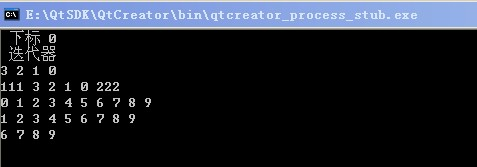（4）数组转置 (<algorithm> reverse)

reverse(v.begin(),v.end())

`````` 1 #include<iostream>
2 #include<vector>
3 #include<algorithm>
4
5 using namespace std;
6
7 int main()
8 {
9     vector<int> v;
10     for(int i = 0; i < 10; ++i)
11     {
12         v.push_back(i);
13     }
14     for(vector<int>::iterator it = v.begin(); it != v.end(); ++it)
15     {
16         cout << *it << " ";
17     }
18     cout << endl;
19
20     reverse(v.begin(),v.end());
21
22
23     for(vector<int>::iterator it = v.begin(); it != v.end(); ++it)
24     {
25         cout << *it << " ";
26     }
27     cout << endl;
28     return 0;
29 }``````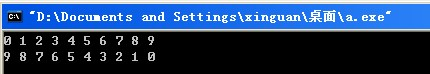（5）排序（<algorithm> sort）

sort(v.begin(),v.end()）

`````` 1 #include<iostream>
2 #include<vector>
3 #include<algorithm>
4
5 using namespace std;
6
7 bool Comp(const int &a,const int &b)
8 {
9     return a>b;
10 }
11
12 int main()
13 {
14     vector<int> v;
15     v.push_back(1);
16     v.push_back(3);
17     v.push_back(2);
18     v.push_back(55);
19     v.push_back(-1);
20     v.push_back(0);
21     v.push_back(2);
22     v.push_back(3);
23     v.push_back(4);
24
25     for(vector<int>::iterator it = v.begin(); it != v.end(); ++it)
26     {
27         cout << *it << " ";
28     }
29     cout << endl;
30
31     //默认升序
32     sort(v.begin(),v.end());
33
34
35     for(vector<int>::iterator it = v.begin(); it != v.end(); ++it)
36     {
37         cout << *it << " ";
38     }
39     cout << endl;
40
41     //用降序 需要自定义一个降序函数
42     sort(v.begin(),v.end(),Comp);
43
44
45     for(vector<int>::iterator it = v.begin(); it != v.end(); ++it)
46     {
47         cout << *it << " ";
48     }
49     cout << endl;
50
51     return 0;
52 }``````

（6）字符串（<string>）

输入

`````` 1 #include<iostream>
2 #include<string>
3 #include<cstdio>
4
5 using namespace std;
6
7 int main()
8 {
9     string s1;
10     s1 = "hello";
11
12     string s2;
13     char s;
14     //scanf 输入速度比cin快的多
15     //scanf 是C函数，不可以输入string
16     scanf("%s",s);
17     s2 = s;
18
19     cout << s1 << endl;
20     cout << s2 << endl;
21
22     return 0;
23 }``````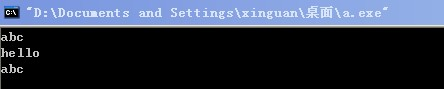（7）删除 （erase clear）

s.erase(it + 1,it + 4); clear（）

`````` 1 #include<iostream>
2 #include<string>
3
4 using namespace std;
5
6 int main()
7 {
8     string s;
9     s = "0123456789";
10     cout << s << endl;
11
12     string::iterator it = s.begin();
13
14     //删除s
15     s.erase(it+3);
16     cout << s << endl;
17
18     //删除s~s
19     s = "0123456789";
20     s.erase(it + 1,it + 4);
21     cout << s << endl;
22
23     //全部删除
24     s.clear();
25     cout << "clear : " << s << endl;
26
27     return 0;
28 }``````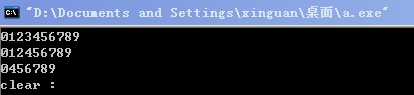（8）查找（find）

用find找到string里面第一个要找到元素（char或者串），找到返回数组下标，找不到返回end（）迭代器

string和vector有很多相同的东西，比如length（），size（），empty（），reverse（），相对也容易，就不一一说了。

（9）数字化处理（string）

经常会遇到这样一种情况，有一个数字，需要把每一位给提取出来，如果用取余数的方法，花费的时间就会很长，所以可以当成字符串来处理，方便、省时。

例子：求一个整数各位数的和

`````` 1 #include<iostream>
2 #include<string>
3
4 using namespace std;
5
6 int main()
7 {
8     string s;
9     s = "123456789";
10     int sum = 0;
11     for(int i = 0; i < s.size(); ++i)
12     {
13         switch(s[i])
14         {
15             case '1': sum += 1;break;
16             case '2': sum += 2;break;
17             case '3': sum += 3;break;
18             case '4': sum += 4;break;
19             case '5': sum += 5;break;
20             case '6': sum += 6;break;
21             case '7': sum += 7;break;
22             case '8': sum += 8;break;
23             case '9': sum += 9;break;
24         }
25     }
26
27     cout << sum << endl;
28
29     return 0;
30 }``````

（10）string与char *

`````` 1 #include<iostream>
2 #include<string>
3 #include<cstdio>
4
5 using namespace std;
6
7 int main()
8 {
9     string s_string;
10     char s_char;
11     scanf("%s",s_char);
12
13     s_string = s_char;
14
15     //printf输出char* 时用c_str处理
16     printf(s_string.c_str());
17     cout << endl;
18
19     printf("%s",s_char);
20     cout << endl;
21
22     cout << s_char << endl;
23     cout << s_string << endl;
24     return 0;
25 }``````

（11）sscanf

`````` 1 #include<iostream>
2 #include<string>
3 #include<cstdio>
4
5 using namespace std;
6
7 int main()
8 {
9     string s1,s2,s3;
10     char sa,sb,sc;
11     sscanf("abc 123 wcd","%s%s%s",sa,sb,sc);
12     s1 = sa;
13     s2 = sb;
14     s3 = sc;
15     cout << s1 << " " << s2 << " " << s3 << endl;
16
17     //将字符串分离成数字，分隔符为',''\$'
18     int a,b,c;
19     sscanf("4,5\$6","%d,%d\$%d",&a,&b,&c);
20     cout << a << " " << b << " " << c << endl;
21     return 0;
22 }``````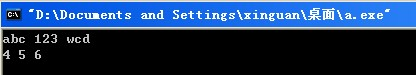（12）string与数值相互转换( sprintf <sstream> )

`````` 1 #include<iostream>
2 #include<string>
3 #include<sstream>
4 #include<cstdio>
5
6 using namespace std;
7
8 //c++ 方法 把数转换为string
9 string converToString(double x)
10 {
11     ostringstream o;
12     if( o << x)
13     {
14         // str()没有'\0' c_str有
15         return o.str();
16     }
17     return "error";
18 }
19
20 double converFromString(const string &s)
21 {
22     istringstream i(s);
23     double x;
24     if( i >> x)
25     {
26         return x;
27     }
28     //if error
29     return 0.0;
30 }
31 int main()
32 {
33     char b;
34     string s1;
35
36     //c语言方法
37     sprintf(b,"%d",1987);
38     s1 = b;
39     cout << s1 << endl;
40
41     string s2 = converToString(1954);
42     cout << s2 << endl;
43
44     string s3 = "202";
45     int c = converFromString(s3);
46     cout << c << endl;
47
48     string s4 = "casacsa6";
49     int d = converFromString(s4);
50     cout << d << endl;
51
52     string s5 = "21abf4";
53     int f = converFromString(s5);
54     cout << f << endl;
55
56     return 0;
57 }``````

https://www.cnblogs.com/CnZyy/p/3317999.html

0
0 收藏

### 作者的其它热门文章0 评论
0 收藏
0